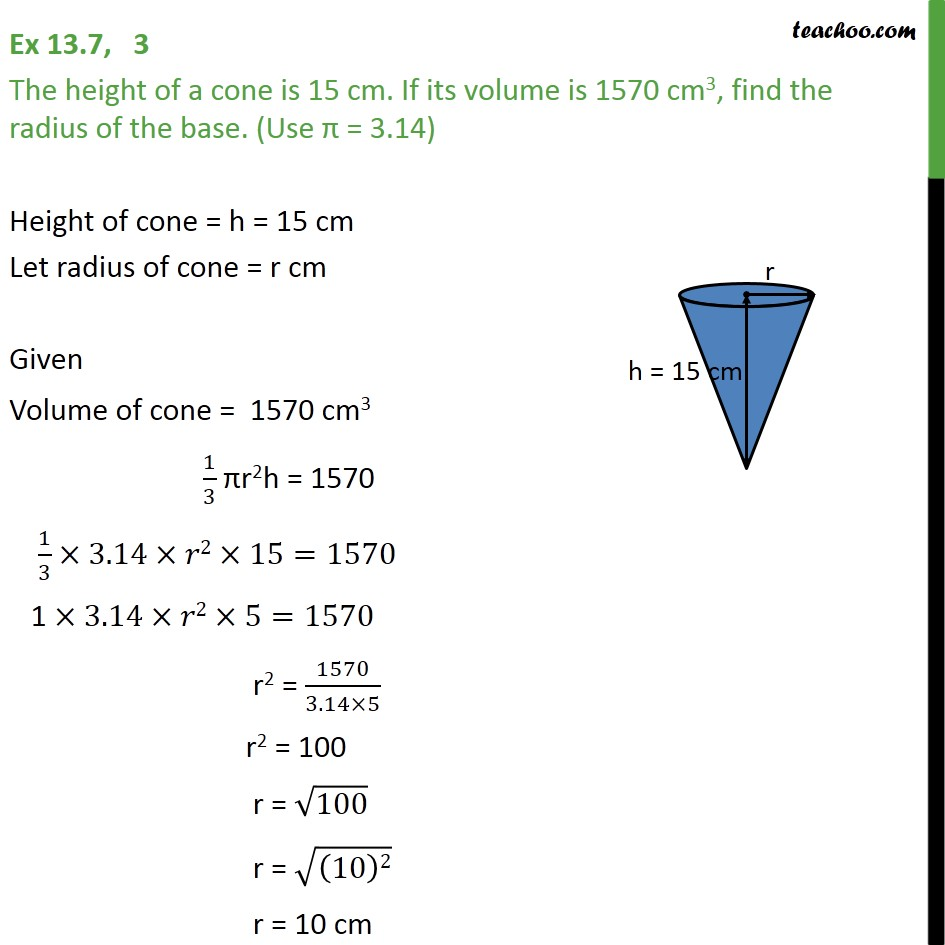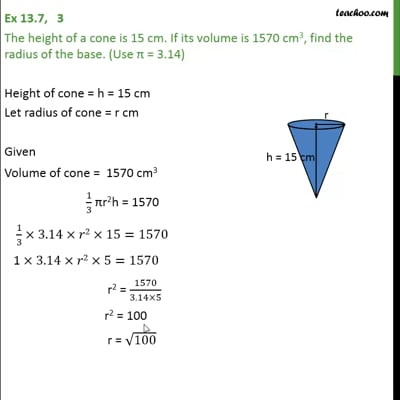Volume Of Cone

Chapter 13 Class 9 Surface Areas and Volumes
Concept wiseThis video is only available for Teachoo black users

Introducing your new favourite teacher - Teachoo Black, at only ₹83 per month

### Transcript

Ex 13.7, 3 The height of a cone is 15 cm. If its volume is 1570 cm3, find the radius of the base. (Use π = 3.14) Height of cone = h = 15 cm Let radius of cone = r cm Given Volume of cone = 1570 cm3 1/3 πr2h = 1570 1/3 ×3.14×𝑟2×15=1570 1 ×3.14×𝑟2×5=1570 r2 = 1570/(3.14×5) r2 = 100 r = √100 r = √((10)2) r = 10 cm